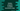# Python program to check if an array is monotonic or not## Monotonic array in Python :

In this python programming tutorial, we will learn how to find if an array is monotonic or not. An array is called monotonic if its adjacent numbers are either increasing or decreasing. Monotonic arrays are divided into two categories: monotone increasing and monotone decreasing.

## Monotone increasing array :

An array is called monotone increasing if for all elements of the array with index_ i_ and_ j,_ array[i] <= array[j] for all i <= j. For example, [1,2,3,4,5] is a_ monotone increasing_ array.

## Monotone decreasing array :

A monotone decreasing array is exactly the opposite of monotone increasing. If for all elements of the array with index_ i_ and_ j,_ array[i] >= array[j], it is called a monotone decreasing array. For example [55,33,22,11] is a monotone decreasing array.

## Python example program to check if an array is monotonic or not :

Let’s write one python program to verify if an array is monotonic or not. Our program will first ask the user to enter the total count of the array. It will then take the array inputs from the user one by one. Finally, it will print out the result to the user.

``````#1
user_array = list()

#2
def is_monotonic(arr):
#3
if all(arr[i] <= arr[i + 1] for i in range(len(arr) - 1)): return "monotone increasing" elif all(arr[i] >= arr[i + 1] for i in range(len(arr) - 1)):
return "monotone decreasing"
return "not monotonic array"

#4
size = int(input("Enter the size of the array : "))

#5
for i in range(size):
n = int(input("Enter value for position {} : ".format(i)))
user_array.append(n)

#6
print("Input array is "+is_monotonic(user_array))``````

### Sample Output :

``````Enter the size of the array : 3
Enter value for position 0 : 1
Enter value for position 1 : 12
Enter value for position 2 : 144
Input array is monotone increasing

Enter the size of the array : 4
Enter value for position 0 : 45
Enter value for position 1 : 33
Enter value for position 2 : 24
Enter value for position 3 : 11
Input array is monotone decreasing

Enter the size of the array : 5
Enter value for position 0 : 1
Enter value for position 1 : 22
Enter value for position 2 : 25
Enter value for position 3 : 55
Enter value for position 4 : 5
Input array is not monotonic array``````

### Explanation :

The commented numbers in the above program denote the step numbers below :

1. Create one list userarray_ to hold all user input values.
2. ismonotonic_ method takes one list and returns one string: “not monotonic array if the input list is not monotonic, “monotone increasing” if the input array is monotone increasing and “monotone decreasing” if the input array is monotone decreasing.
3. Inside this method, using one if-elif-else condition, it is checked if all elements in the given list are continuously increasing, continuously decreasing or not. Based on the condition, it returns one string as explained above.
4. This line is the start point of the program. It will ask the user to enter the size of the array and store it in size variable.
5. Using one for loop, it will continuously ask the user to enter elements for the array. Each element is appended to the list userarray_.
6. Finally, the program will call the ismonotonic_ method and prints the result.

### Conclusion :

In this example, we are returning_ three different strings_ after checking the list if it is monotonic or not. Instead of returning a string, you can also return three different integers and print the result based on the return value. Try to run the program and if you have any query, don’t hesitate to drop one comment below.